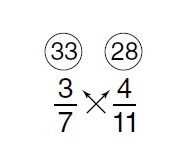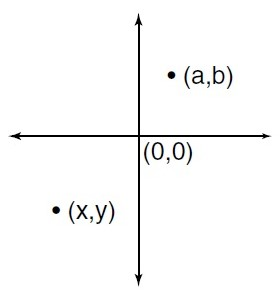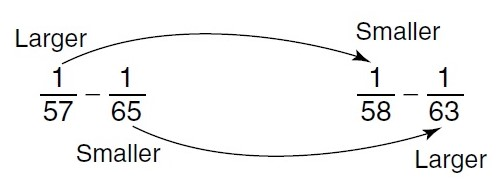In the name of ALLAH, the most beneficient, the most merciful

# Quantitative Comparison

Quantitative Comparison questions require you to make a comparison between quantities in two columns. You are to decide if one column is greater, if the columns are equal, or if no comparison can be determined from the information given.

Quantitative Comparison tests your ability to use mathematical insight, approximation, simple calculation, or common sense to quickly compare two given quantities.

This question type requires twelfth-grade competence in school arithmetic, algebra, and intuitive geometry. Skills in approximating, comparing, and evaluating are also necessary. No advanced mathematics is necessary.

## Directions

You are given two quantities, one in column A and one in column B. You are to compare the two quantities and choose oval:

1. if the quantity in Column A is greater;
2. if the quantity in Column B is greater;
3. if the two quantities are equal;
4. if the comparison cannot be determined from the information given.

Common Information: Information centered above columns refers to one or both columns. A symbol that appears in both columns represents the same thing in each column.

## Analysis of Directions

• The purpose here is to make a comparison; therefore, exact answers are not always necessary. (Remember that you can tell whether you are taller than someone in many cases without knowing that person’s height. Comparisons such as this can be made with only limited or partial information-just enough to compare.)
• Choice D-the comparison cannot be determined from the information given-is not a possible answer if there are values in each column, because you can always compare values.
• If you get different relationships, depending on the values you choose for variables, then the answer is always D. Notice that there are only four possible choices here.
• Note that you can add, subtract, multiply, and divide both columns by the same value, and the relationship between the columns will not change. Exception: You should not multiply or divide each column by negative numbers, because the relationship reverses. Squaring both columns is permissible, as long as each side is positive.

## Suggestions with Examples

This section emphasizes shortcuts, insight, and quick techniques. Long and/or involved mathematical computation is unnecessary and is contrary to the purpose of this section.
Column A Column B
21 × 43 × 56 44 × 21 × 57

Canceling (or dividing) 21 from each side leaves

 43 × 56 44 × 57

The rest of this problem should be done by inspection, because it is obvious that column B is greater than column A without doing any multiplication. You could have attained the correct answer by actually multiplying out each column, but you would then not have enough time to finish the section. The correct answer is B.

Column A Column B
3/7 × 2/5 × 5/8 2/5 × 4/11 × 5/8

Because both sides have the factors 2⁄5 and 5⁄8, you may eliminate them from each column.

 3/7 × 2/5 × 5/8 2/5 × 4/11 × 5/8

Now compare 3⁄7 and 4⁄11 by cross-multiplying upward, and you getBecause 33 is greater than 28, 3⁄7 > 4⁄11. The correct answer is A.

Always keep the columns in perspective before starting any calculations. Take a good look at the value in each column before starting to work on one column.
Column A Column B
40% of 60 60% of 40

There is no need to do any calculations for this problem. Column A can be written (40⁄100) × 60. Column B can be written (60⁄100) × 40. You should note that both columns have (40 × 60)/100. The correct answer is C.

Column A Column B
∛76 28

After looking at each column (note that the answer could not be D because there are values in each column), compute the value on the left. Because you are taking a cube root, simply divide the power of 7 by 3 leaving 72, or 49. There is no need to take 2 out to the 8th power; just do as little as necessary:

22 = 4
23 = 8
24 = 16
25 = 32
STOP

It is evident that 28 is much greater than 49; the correct answer is B. Approximating can also be valuable while remembering to keep the columns in perspective.

As you keep the columns in perspective, check to see if the value in each column increases or decreases from the starting point.
Column A Column B
(.9)8 (1.01)4

In Column A, a fractional value (a value less than 1) is multiplied by itself many times. So its value becomes increasingly smaller. (For example, 1⁄2 × 1⁄2 = 1⁄4; 1⁄4 × 1⁄2 = 1⁄8, and so forth). In Column B, a number greater than 1 is multiplied by itself; its value grows larger. So Column B is greater. The correct answer is B.

As you keep the columns in perspective, notice if the signs (+, −) in each column are different. If they are, you don’t need to work out the problem.
Column A Column B
(−10)100 (−10)101

A negative number multiplied an even number of times will yield a positive product. A negative number multiplied an odd number of times will yield a negative product. Since Column A will be positive and Column B will be negative, A is greater. The correct answer is A.

Column A Column B
.05 − .125 .1

Subtracting in Column A, you get .05 − .125 = −.075. Our difference is a negative number. Thus, the positive value in Column B must be greater. The correct answer is B.

Column A Column Bx + y a + b

Because coordinates (x,y) are in quadrant III, they are both negative, so their sum is negative. Because coordinates (a, b) are in quadrant I, they are both positive, so their sum is positive. Therefore, Column B is greater than Column A. The correct answer is B.

The use of partial comparisons can be valuable in giving you insight into finding a comparison. If you cannot simply make a complete comparison, look at each column part by part.
Column A Column B
1/57 - 1/65 1/58 - 1/63

Because finding a common denominator would be too time consuming, you should first compare the first fraction in each column (partial comparison). Notice that 1⁄57 is greater than 1⁄58. Now compare the second fractions and notice that 1⁄65 is less than 1⁄63. Using some common sense and insight, if you start with a larger number and subtract a smaller number, it must be greater than starting with a smaller number and subtracting a larger number, as pointed out below.Often, simplifying one or both columns can make an answer evident.
Column A Column B
a, b, c, all greater than 0
a(b + c) ab + ac

Using the distributive property on Column A to simplify gives ab and ac; therefore, the columns are equal. The correct answer is C.

Column A Column B
a > 0
b > 0
c > 0
(3a)(3b)(3c) 3abc

Multiplying column A gives (3a)(3b)(3c) = 27abc. Because a, b, and c are all positive values, 9abc will always be greater than 3abc. The correct answer is A.

Column A Column B
Number of prime numbers between 3 and 19 5

The prime numbers between 3 and 19 are 5, 7, 11, 13, and 17. The correct answer is C, since there are 5 primes.

If a problem involves variables (without an equation), substitute in the numbers 0, 1, and -1. Then try 1⁄2, and 2 if necessary. Using 0, 1, and -1 will often tip off the answer.
Column A Column B
a + b ab

Substituting 0 for a and 0 for b gives the following:

 0 + 0 (0)

Therefore, 0 = 0.

Using these values for a and b gives the answer C. But when you multiply two numbers, you don’t always get the same result as when you add them, so try some other values. Substituting 1 for a and −1 for b gives the following:

 1 + (−1) 1(−1)

Therefore, 0 > −1 and the answer is now A.

Anytime you get more than one comparison (different relationships), depending on the values you choose, the correct answer must be D, the relationship cannot be determined. Notice that if you had substituted the values a = 4, b = 5; or a = 6, b = 7; or a = 7, b = 9; and so on, you would repeatedly get the answer B and may have chosen the incorrect answer. The correct answer is D.

Column A Column B
x < y < z
x + y + z xyz

Substituting 0 for x, 1 for y, and 2 for z, gives

 (0) + (1) + (2) (0)(1)(2)

Therefore, 3 > 0.

Now substituting −1 for x, 0 for y, and 1 for z gives

 (−1) + (0) + (1) (−1)(0)(1)

Therefore, 0 = 0.

Because different values give different comparisons, the correct answer is D.

Column A Column B
x > y > 0
x and y are integers
((x + y)x)/x ((x + y)y)/y

Plug in values for x and y such that x > y > 0, and x and y are integers. For example, let y = 1 and x = 2. This gives

 ((2 + 1)2)/2 ((1 + 1)1)/1 (3)2/2 (2)1/1
 9/2 > 3/1

Using these values, 9⁄2, or 4(1⁄2), is greater than 3, so Column A is greater. Using other values such that x > y > 0 will always give the same relationship. Column A is greater. The correct answer is A.

Sometimes you can solve for a column directly, in one step, without solving and substituting. If you have to solve an equation or equations to give the columns values, take a second and see if there is a very simple way to get an answer before going through all of the steps.
Column A Column B
4x + 2 = 10
2x + 1 4

Hopefully, you would spot that the easiest way to solve for 2x + 1 is directly by dividing 4x + 2 = 10 by 2, leaving 2x + 1 = 5. Therefore, 5 > 4
Solving for x first in the equation and then substituting would also have worked but would have been more time consuming. The correct answer is A.

Redrawing and marking diagrams and figures can be very helpful for giving insight into a problem. If you are given a diagram or figure on the screen, quickly redraw it on your scratch paper. Remember that diagrams and figures are meant for positional information only. Just because something "looks" a certain way is not enough reason to choose an answer.
Column A Column Bx y

Even though x appears larger, this is not enough. Mark in the diagram as shown.Notice that you should mark things of equal measure with the same markings, and since angles opposite equal sides in a triangle are equal, x = y. The correct answer is C.

If you are given a description of a diagram or a geometry problem without a diagram, you should make a sketch. When in doubt, “draw.” This may tip off a simple solution.
Column A Column B
Perimeter of an equilateral triangle with side length 5x Perimeter of a square with side length of 4x

Simply sketch and label each geometric figure as follows:Now it is evident that the perimeter of an equilateral triangle with side 5x is
3(5x) = 15x
The perimeter of a square with side 4x is 4(4x) = 16x.
Since 4x and 5x represent lengths of sides, x must be a positive number. Therefore,
15x < 16x

If you are given information that is unfamiliar to you and difficult to work with, change the number slightly (but remember what you’ve changed) to something easier to work with.
Column A Column B5 c

Becasue the 88° shown in the figure is unfamiliar to work with, change it to 90° for now so that you may use the Pythagorean theorem to solve for c.

a2 + b2 = c2

Solve for c as follows:

(3)2 + (4)2 = c2
9 + 16 = c2
25 = c2

Therefore, 5 = c.

But because you used 90° instead of 88°, you should realize that the side opposite the 88° will be slightly smaller, or less than 5. The correct answer is then A, 5 > c. (You may have noticed the 3:4:5 triangle relationship and not have needed the Pythagorean theorem.)

Check to see if you actually have enough information to make a comparison. Be aware of the information you are given and what you need.
Column A Column B
Distance traveled by an airplane going 200 miles per hour Distance traveled by an airplane going 190 miles per hour

Because no information is given for the amount of time each of the airplanes was traveling, no determination can be made about their distances traveled. You cannot assume that each airplane traveled the same amount of time. The correct answer is D.

Column A Column B
Volume of right circular cylinder with diameter of x Volume of right circular cylinder with diameter of x + 1

The correct answer is D. Because the height of each right circular cylinder is necessary to obtain the volume, no comparison can be made.

On occasion, you will actually have to solve information centered between the columns or information in the columns. You should be able to work these quickly. Remember, if it takes too long, you’re probably doing it wrong.
Column A Column B
The value of 5x − 9 when x = 6 The value of 9 − 5x when x = −6

Quickly plug in the values given.

 5x −9 9 − 5x 5(6) −9 9 − 5(−6) 30 −9 9 + 30(−6)
 21 < 39

Therefore, B is the correct answer.

Column A Column B
m and n are integers.
0 < m < n < 5
mn m + n

After taking a careful look at the center information, you realize that you’re limited in the numbers that you can use for m and n. Try some possibilities within these limitations.

Substituting 1 for m and 2 for n gives

 1(2) 1 + 2
 2 < 3

So when you use these numbers, Column B is greater.

Now try some other possible numbers. Substituting 3 for m and 4 for n, you get

 3(4) 3 + 4
 12 > 7

So when you use these numbers, Column A is greater.

The correct answer is D, no comparison can be determined.

Remember, anytime you get more than one comparison (different relationships) depending on the values you choose, the correct answer is D, the relationship cannot be determined.